### Related Articles

Google Apps | Tutorial

Page 2 of 2

### Google Sheets: Using Custom Formulas in Conditional Formatting

In a previous Google Apps tutorial, we looked at a method for formatting spreadsheet cells based on a date. If a date were X days in the future, the cell and text would appear in a certain color. If it were Y days in the future, they would be a different color.

Then a reader wrote to me asking if it would be possible to exclude weekends when making such calculations. In other words, can we format a cell conditionally using only school days, business days or work days as a reference?

Yes.

Within the Conditional Formatting function, Google has provided the option of using custom formulas. These custom formulas are what you'll have to use in order to make this thing work.

NETWORKDAYS
Weekdays (school days, business days) are calculated using the NETWORKDAYS. If you want to count the number of net working days between today and a week from today (inclusive of today), you could simply enter:

=NETWORKDAYS(TODAY(),TODAY()+7)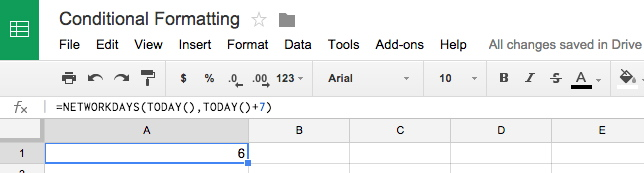This should yield an answer of 6 if today is a weekday, 5 if today is a weekend day.

We can also use this feature in conditional formatting. But we'll need to do quite a bit more work on it first.

IF
Using IF, we can get Google sheets to return one value if a statement is true and another if the statement is false. To help you understand how this works conceptually, try plugging this into a cell in one of your spreadsheets:

=if(NETWORKDAYS(TODAY(),TODAY()+7)=6,"Today is a weekday","Today is a weekend day")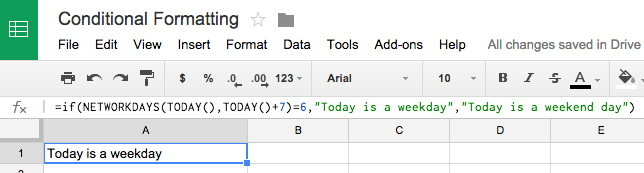That's a simple test to determine if today is a weekday or not. The formula simply says if the number of net working days from today to seven days from today is 6, then display "Today is a weekday"; if not, display "Today is a weekend day." (Note: If you plan to output text, the text must appear within quotation marks in this expression.)

The answer will change depending on which day you view your spreadsheet, since we used the dynamic value "TODAY()."

You can also used fixed dates in the same way:

=if(NETWORKDAYS(DATE(2014,9,22),DATE(2014,9,29))=6,"Today is September 22, 2014","You typed something wrong")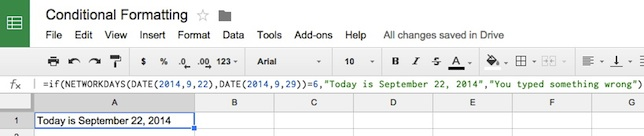That particular one will always display "Today is September 22, 2014" ... unless you typed something wrong in the formula. (As I began writing this, the date was, in fact, Sept. 22, 2014.)

In addition to =, you can also use < and > in your if statements.

=if(NETWORKDAYS(TODAY(),TODAY()+7)<6,"Today is a weekend day","Today is a weekday")

So: If the number of days from today to a week from today is less than 6, today is a weekend; otherwise it's a weekday.

And, importantly for today's tutorial, you can also output the result as a number, instead of a string of text.

=if(NETWORKDAYS(TODAY(),TODAY()+7)<6,1,0)

Here 1 will be displayed if the statement is true; 0 will be displayed if it's false.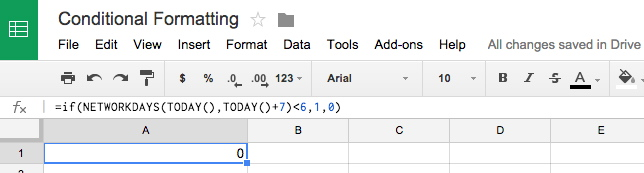One other crucial ingredient: You can also reference the date contained in a cell and measure how many net working days that is from today.

=if(NETWORKDAYS(TODAY(),B2)<6,1,0)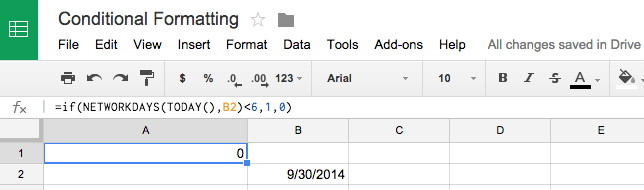That means that if the number of weekdays from today through the date contained in cell B2 is less than 6, a value of 1 will be displayed.

And once we can do that, we can use this type of formula to format a cell conditionally based on the output of "1" or "0."

Thinking Ahead To Save Time
One thing about conditional formatting: It can be very time-consuming to replicate a formula over and over for a given cell and replace, one by one, each reference to a specific cell. In general, formulas in Google Sheets will contextually update themselves when you copy from one cell and paste to another. But that doesn't happen with formulas used in conditional formatting. So in our formula, we want to do that dynamically.

A cell can by called dynamically by first selecting an entire column, then using the INDIRECT function.

If I'm formatting column B, for example, INDIRECT would look like this:

INDIRECT("B"&ROW())

So if we substitute that into our previous formula, replacing "B2," we get:

=if(NETWORKDAYS(TODAY(),(INDIRECT("B"&ROW())))<6,1,0)

Translation: If the number of working days between today and the date contained in any given cell in column B is less than 6, output a 1; if it isn't, output a zero.

Phew!

Putting It All Together
But now we need to put this together into something that Google's Conditional Formatting will understand.

Select your column by clicking on the column name or letter up at the top.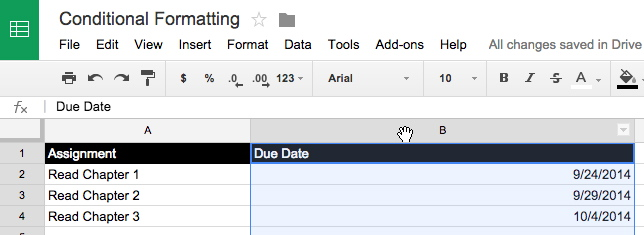Then open up the Conditional Formatting Dialog (Format > Conditional formatting...). Be sure to switch the condition from the default "Text contains" to "Custom formula is."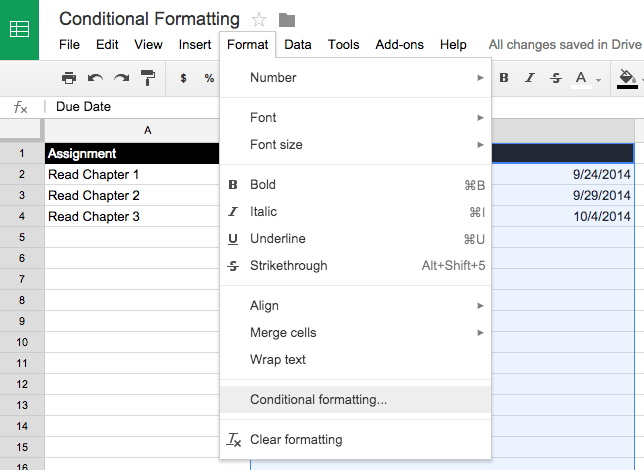For this example, we'll keep it simple and format the cell conditionally based on just three criteria: Is the date contained within the cell more than, less than or equal to six days from today?

First we need to use our formula to get an output result of 1 or 0, then we need to make sure the output is 1 to apply the conditional formatting.

In order to do that, we put the entire "if" statement inside parentheses, then, following the closing parentheses, add "=1" like this:

=(if(NETWORKDAYS(TODAY(),(INDIRECT("B"&ROW())))<6,1,0))=1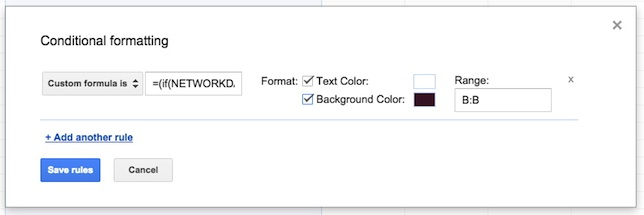Then simply choose your formatting options for when this condition is met, and repeat for the other two conditions (greater than and equal to).

=(if(NETWORKDAYS(TODAY(),(INDIRECT("B"&ROW())))>6,1,0))=1

=(if(NETWORKDAYS(TODAY(),(INDIRECT("B"&ROW())))=6,1,0))=1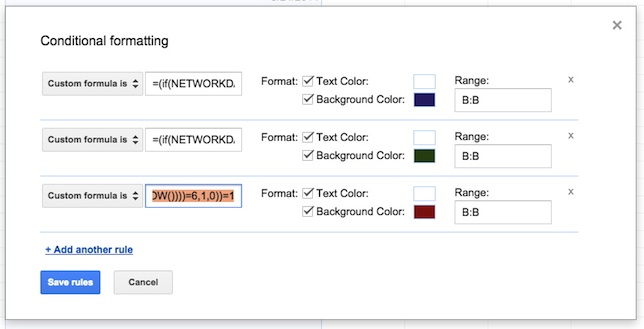Click "Save Rules" in the dialog, and voila!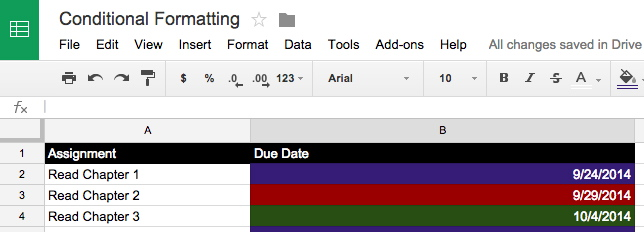You are now calculating the number of weekdays from now through any date you choose and using the result to format each cell individually.

Looking for more tutorials? Check out our Tutorial archive!David Nagel is editorial director of 1105 Media's Education Technology Group and editor-in-chief of THE Journal and STEAM Universe. A 25-year publishing veteran, Nagel has led or contributed to dozens of technology, art and business publications.

He can be reached at dnagel@1105media.com. You can also connect with him on LinkedIn at or follow him on Twitter at @THEJournalDave (K-12) or @CampusTechDave (higher education).